Learn how to program games with the LÖVE framework

# Chapter 18 - Tiles

Be sure to read the comments in the code, I put a lot of important information in there

In a lot of 2D games the levels are made out of tiles. We're going to make our own tiled level.

Let's start off by creating a line. Create a table and fill it with ones and zeroes.

``````function love.load()
tilemap = {1, 0, 0, 1, 1, 0, 1, 1, 1, 0}
end``````

This is our level. A `1` is a tile and a `0` is empty. Now we need to draw it. We loop through the table, and every time we encounter a 1, we draw a rectangle on its position.

``````function love.draw()
--ipairs recap
--ipairs is a special function that allows you to loop through a table
--Every iteration i becomes what iteration the loop is at, so 1, 2, 3, 4, etc)
--Every iteration v becomes the value on position i, so in our case 1, 0, 0, 1, 1, 0, etc.
for i,v in ipairs(tilemap) do
if v == 1 then
love.graphics.rectangle("line", i * 25, 100, 25, 25)
end
end
end``````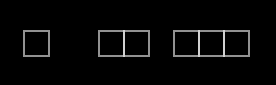Okay so this works, but now we want to go vertical. We do this by putting tables inside a table, also known as a 2D table.

``````function love.load()
tilemap = {
{1, 1, 1, 1, 1, 1, 1, 1, 1, 1},
{1, 0, 0, 0, 0, 0, 0, 0, 0, 1},
{1, 0, 0, 1, 1, 1, 1, 0, 0, 1},
{1, 0, 0, 0, 0, 0, 0, 0, 0, 1},
{1, 1, 1, 1, 1, 1, 1, 1, 1, 1}
}
end``````

So now we have a table filled with tables. See it as an Excel table.1, 2, 3, etc. are what we call rows and A, B, C, etc. are called columns.

Another way to look at it as a small town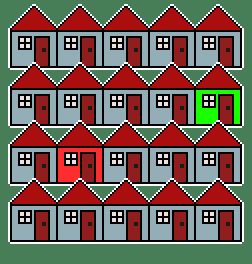Every row of houses is a table, and multiple rows make the whole town, or in our case our level.

The green house is on the 2nd row on the 5th column.

The red house is on the 3rd row on the 2nd column.

With 2D tables we access the values like this:

`tilemap`

This means: The 3rd value of the 4th table. Or: The 3rd column on the 4th row.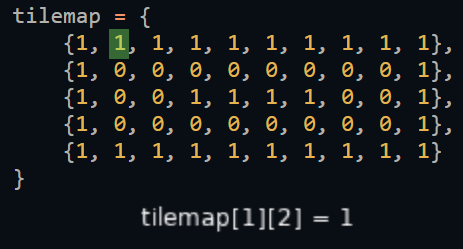Let's draw our level. Because we have a 2D table, we need to use a for-loop inside a for-loop. This is also called a nested for-loop.

``````function love.draw()
--Let's do it without ipairs first.

--For i=1 till the number of values in tilemap
for i=1,#tilemap do
--For j till the number of values in this row
for j=1,#tilemap[i] do
--If the value on row i, column j equals 1
if tilemap[i][j] == 1 then
--Draw the rectangle.
--Use i and j to position the rectangle.
-- j for x, i for y.
love.graphics.rectangle("line", j * 25, i * 25, 25, 25)
end
end
end
end``````

So we loop through our rows, and for every row we loop through our columns.

We use `j`, of our inner for-loop, for our horizontal positioning and `i`, of our outer for-loop for the y positioning. Remember that these are just variable names and can be named whatever, but using i and j like this is common.

Let's turn the for-loops into an ipairs loop.

``````function love.draw()
for i,row in ipairs(tilemap) do
for j,tile in ipairs(row) do
if tile == 1 then
love.graphics.rectangle("line", j * 25, i * 25, 25, 25)
end
end
end
end``````

We use the variable names `row` and `tile` to make it more clear what is going on. We loop through the table `tilemap`, and each value is a row. We loop through the row and each value is a tile.

We can also use different numbers for our tiles, and use these numbers to give the tiles different colors.

``````function love.load()
tilemap = {
{1, 1, 1, 1, 1, 1, 1, 1, 1, 1},
{1, 2, 2, 2, 2, 2, 2, 2, 2, 1},
{1, 2, 3, 4, 5, 5, 4, 3, 2, 1},
{1, 2, 2, 2, 2, 2, 2, 2, 2, 1},
{1, 1, 1, 1, 1, 1, 1, 1, 1, 1}
}
end

function love.draw()
for i,row in ipairs(tilemap) do
for j,tile in ipairs(row) do
--First check if the tile is not zero
if tile ~= 0 then

--Set the color based on the tile number
if tile == 1 then
--setColor uses RGB, A is optional
--Red, Green, Blue, Alpha
love.graphics.setColor(1, 1, 1)
elseif tile == 2 then
love.graphics.setColor(1, 0, 0)
elseif tile == 3 then
love.graphics.setColor(1, 0, 1)
elseif tile == 4 then
love.graphics.setColor(0, 0, 1)
elseif tile == 5 then
love.graphics.setColor(0, 1, 1)
end

--Draw the tile
love.graphics.rectangle("fill", j * 25, i * 25, 25, 25)
end
end
end
end``````

Or a better way to do this:

``````function love.load()
tilemap = {
{1, 1, 1, 1, 1, 1, 1, 1, 1, 1},
{1, 2, 2, 2, 2, 2, 2, 2, 2, 1},
{1, 2, 3, 4, 5, 5, 4, 3, 2, 1},
{1, 2, 2, 2, 2, 2, 2, 2, 2, 1},
{1, 1, 1, 1, 1, 1, 1, 1, 1, 1}
}

--Create a table named colors
colors = {
--Fill it with tables filled with RGB numbers
{1, 1, 1},
{1, 0, 0},
{1, 0, 1},
{0, 0, 1},
{0, 1, 1}
}
end

function love.draw()
for i,row in ipairs(tilemap) do
for j,tile in ipairs(row) do
--First check if the tile is not zero
if tile ~= 0 then
--Set the color. .setColor() also accepts a table with 3 numbers.
--We pass the table with as position the value of tile.
--So if tile equals 3 then we pass colors which is {1, 0, 1}
love.graphics.setColor(colors[tile])
--Draw the tile
love.graphics.rectangle("fill", j * 25, i * 25, 25, 25)
end
end
end
end``````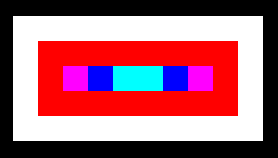## Images

So we can make a colorful level, but now we want to use images. Well that's easy, just add an image, get the width and height, and draw the image instead of a rectangle.

I will use this image:``````function love.load()

image = love.graphics.newImage("tile.png")

--Get the width and height
width = image:getWidth()
height = image:getHeight()

tilemap = {
{1, 1, 1, 1, 1, 1, 1, 1, 1, 1},
{1, 2, 2, 2, 2, 2, 2, 2, 2, 1},
{1, 2, 3, 4, 5, 5, 4, 3, 2, 1},
{1, 2, 2, 2, 2, 2, 2, 2, 2, 1},
{1, 1, 1, 1, 1, 1, 1, 1, 1, 1}
}

colors = {
--Fill it with tables filled with RGB numbers
{1, 1, 1},
{1, 0, 0},
{1, 0, 1},
{0, 0, 1},
{0, 1, 1}
}
end

function love.draw()
for i,row in ipairs(tilemap) do
for j,tile in ipairs(row) do
if tile ~= 0 then
love.graphics.setColor(colors[tile])
--Draw the image
love.graphics.draw(image, j * width, i * height)
end
end
end
end``````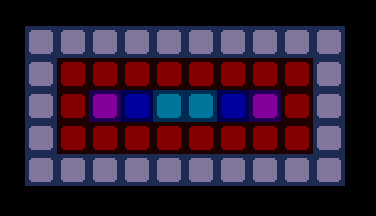So that's easy. But what if we want to draw different images? Well we could use multiple images, but in the previous chapter we learned how we can draw part of an image with quads. We can use this for tiles as well.

Let's use this tileset: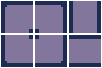First we need to create the quads.

``````function love.load()

image = love.graphics.newImage("tileset.png")

--We need the full image width and height for creating the quads
local image_width = image:getWidth()
local image_height = image:getHeight()

--The width and height of each tile is 32, 32
--So we could do:
width = 32
height = 32
--But let's say we don't know the width and height of a tile
--We can also use the number of rows and columns in the tileset
--Our tileset has 2 rows and 3 columns
--But we need to subtract 2 to make up for the empty pixels we included to prevent bleeding
width = (image_width / 3) - 2
height = (image_height / 2) - 2

for i=0,1 do
for j=0,2 do
--The only reason this code is split up in multiple lines
--is so that it fits the page
1 + j * (width + 2),
1 + i * (height + 2),
width, height,
image_width, image_height))
end
end

tilemap = {
{1, 1, 1, 1, 1, 1, 1, 1, 1, 1},
{1, 2, 2, 2, 2, 2, 2, 2, 2, 1},
{1, 2, 3, 4, 5, 5, 4, 3, 2, 1},
{1, 2, 2, 2, 2, 2, 2, 2, 2, 1},
{1, 1, 1, 1, 1, 1, 1, 1, 1, 1}
}

end``````

Now that we have a table with quads, we put the number in our tilemap based on which quad we want. Based on the order we created our quads, they are on this position in our table: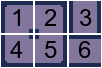So if we wanted to create this: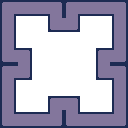We would make our tilemap look like this:

``````tilemap = {
{1, 6, 6, 2, 1, 6, 6, 2},
{3, 0, 0, 4, 5, 0, 0, 3},
{3, 0, 0, 0, 0, 0, 0, 3},
{4, 2, 0, 0, 0, 0, 1, 5},
{1, 5, 0, 0, 0, 0, 4, 2},
{3, 0, 0, 0, 0, 0, 0, 3},
{3, 0, 0, 1, 2, 0, 0, 3},
{4, 6, 6, 5, 4, 6, 6, 5}
}``````

If you compare the tilemap with the image and the numbers you can see how each tile is used.

Now we need to draw the correct quad.

``````function love.draw()
for i,row in ipairs(tilemap) do
for j,tile in ipairs(row) do
if tile ~= 0 then
--Draw the image with the correct quad
love.graphics.draw(image, quads[tile], j * width, i * height)
end
end
end
end``````

So on (1,1) we draw the quad on position 1. On (1,2) we draw the quad on position 6, etc.

If you run the game you'll see that our level now looks like the image above.

## Player

Now that we have a level, let's create a player that can walk around, but not go through walls.

I will use this image for the player:``````function love.load()
image = love.graphics.newImage("tileset.png")

local image_width = image:getWidth()
local image_height = image:getHeight()
width = (image_width / 3) - 2
height = (image_height / 2) - 2

for i=0,1 do
for j=0,2 do
1 + j * (width + 2),
1 + i * (height + 2),
width, height,
image_width, image_height))
end
end

tilemap = {
{1, 6, 6, 2, 1, 6, 6, 2},
{3, 0, 0, 4, 5, 0, 0, 3},
{3, 0, 0, 0, 0, 0, 0, 3},
{4, 2, 0, 0, 0, 0, 1, 5},
{1, 5, 0, 0, 0, 0, 4, 2},
{3, 0, 0, 0, 0, 0, 0, 3},
{3, 0, 0, 1, 2, 0, 0, 3},
{4, 6, 6, 5, 4, 6, 6, 5}
}

--Create our player
player = {
image = love.graphics.newImage("player.png"),
tile_x = 2,
tile_y = 2
}
end``````

The `tile_x` and `tile_y` is the player's position on our tilemap. This number will be multiplied by the tile width and height when drawn. But first let's make it move. Instead of smooth movement we will make it jump to its next position, so we won't be needing `dt` for the movement. This also means that we don't want to know if the movement keys are down, but if they are pressed. For this we use the `love.keypressed` event.

First we create local x and y variable. Next we add or subtract 1 to this variable based on the key that was pressed, and finally we assign this value to the player's position.

``````function love.keypressed(key)
local x = player.tile_x
local y = player.tile_y

if key == "left" then
x = x - 1
elseif key == "right" then
x = x + 1
elseif key == "up" then
y = y - 1
elseif key == "down" then
y = y + 1
end

player.tile_x = x
player.tile_y = y
end``````

Now that it can move, let's draw it.

``````function love.draw()
for i,row in ipairs(tilemap) do
for j,tile in ipairs(row) do
if tile ~= 0 then
--Draw the image with the correct quad
love.graphics.draw(image, quads[tile], j * width, i * height)
end
end
end

--Draw the player and multiple its tile position with the tile width and height
love.graphics.draw(player.image, player.tile_x * width, player.tile_y * height)
end``````When you run the game you should be able to walk around with your player. But the problem is that he can walk through walls. Let's fix this by checking if the position he wants to go to is a wall.

First make a function called `isEmpty`. Inside we return whether the value on the coordinates equals 0.

``````function isEmpty(x, y)
return tilemap[y][x] == 0
end``````

It might look weird that x and y are turned around, but this is correct. Because the y position is the row and the x position is the column.

Now that we have our function we can check if where we want to go is an empty spot, and if so it means we can walk.

``````function love.keypressed(key)
local x = player.tile_x
local y = player.tile_y

if key == "left" then
x = x - 1
elseif key == "right" then
x = x + 1
elseif key == "up" then
y = y - 1
elseif key == "down" then
y = y + 1
end

if isEmpty(x, y) then
player.tile_x = x
player.tile_y = y
end
end``````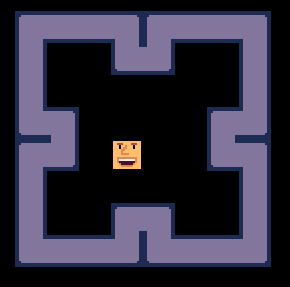Yay, now our player is trapped inside our walls. Try to see if you can make it pick things up, or have it open a door when you touch a key. Play around with this because that is how you learn.

## Summary

We can use tiles to make levels. A tilemap is made out of rows and columns. Each row contains a number of columns. Rows are lined up vertically and columns horizontally. We can use a tileset and quads to draw our level.

Do you need help or do you see a mistake?